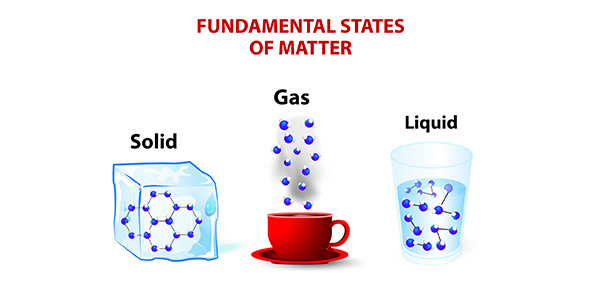# Matter And Its Properties

20 QuestionsSettingsTest 2; Matter and its Properties

Related Topics
• 1.
The density of an object is a ---
• A.

Chemical Property

• B.

Combustible Property

• C.

Physical Property

• D.

Reactive Property

• 2.
A pure substance always has ______ desisty when measured under _____ conditions.
• A.

The same; different

• B.

The same; the same

• C.

A different; the same

• D.

A different; different

• 3.
A solution is a type of--
• A.

Matter

• B.

Mixture

• C.

Balance

• D.

Combustibility

• 4.
Weight is measured on a scale. Mass is measured on a --
• A.

Sale

• B.

Barometer

• C.

Balance

• D.

Thermometer

• 5.
Which of the following does Not signal a chemical change?
• A.

Change in state

• B.

Production of gas

• C.

Color change

• D.

Production of light and heat

• 6.
Making skim milk is an example of ---
• A.

Combustibility

• B.

Solubility

• C.

Using physical properties to separate a mixture

• D.

Using chemical properties to seperate a mixture

• 7.
A person's _____ is different on a high mountain than it is at sea level.
• A.

Chemical property

• B.

Density

• C.

Mass

• D.

Weight

• 8.
Two similar looking substances are burned. One produces a red flame, and the other produces a yellow flame. This is an example of ---
• A.

A flame test

• B.

An indicator test

• C.

A combustibility test

• D.

A reactivity test

• 9.
Given a solution of sugar and water, and water and pepper, which is true.
• A.

If stired, the pepper would have the same solubility as sugar.

• B.

Pepper is soluble in water, bur sugar is not.

• C.

Sugar is soluble in water, but pepper is not.

• D.

Sugar and peper have the same solubility

• 10.
Other ____of substance, like color, size, and hardness, can be found without changing the substance into something else.
• A.

Reactivity

• B.

Physical properties

• C.

Evaporation

• D.

Chemical Properties

• 11.
An object's ____ is the pull of gravity on the object
• A.

Solubility

• B.

Mass

• C.

Weight

• D.

Volume

• E.

Matter

• 12.
A ____ has a definate volume but no definite shape.
• A.

Solid

• B.

Liquid

• C.

Gas

• D.

Matter

• E.

Density

• 13.
A ____ does not have a definite volume or a definite shape.
• A.

Gas

• B.

Solid

• C.

Liquid

• D.

Volume

• E.

Weight

• 14.
A _____ has both definite volume and shape.
• A.

Liquid

• B.

Solid

• C.

Weight

• D.

Gas

• E.

Matter

• 15.
You can divide the mass of an object by its volume to find the object's ______.
• A.

Physical properties

• B.

Solubility

• C.

Reactivity

• D.

Density

• E.

Volume

• 16.
All material that takes up space is called.
• A.

Mass

• B.

Matter

• C.

Solubility

• D.

Weight

• E.

Volume

• 17.
The amount of material in an object is called the object's _____.
• A.

Physical properties

• B.

Density

• C.

Volume

• D.

Weight

• E.

Mass

• 18.
You can measure the amount of space a solid object takes up, or the _____ of a solid object, by placing the object in a graduated cylinder with water and measuring how much water is displaced.
• A.

Mass

• B.

Density

• C.

Volume

• D.

Weight

• E.

Solubility

• 19.
Chemical changes can turn substances into other substances. The ability of a substance to go through a chemical change is called _____.
• A.

Evaporation

• B.

Physical properties

• C.

Combustibility

• D.

Reactivity

• E.

Condensation

• 20.
Sometimes the ability to be dissolved, or ________, can be used to identify a substance.
• A.

Solubility

• B.

Matter

• C.

Volume

• D.

Liquid

• E.

Gas